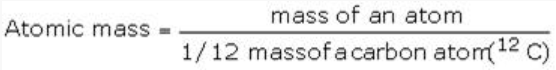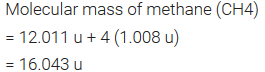# Dalton’s Atomic Theory

The origin of the idea that matter is composed of small indivisible particles called ‘a-Tomio’ (meaning — indivisible). In 1808, Dalton published ‘A New System of Chemical Philosophy’ in which he proposed the following:

• Matter consists of indivisible atoms.
• All the atoms of a given element have identical properties including identical mass. Atoms of different elements differ in mass.
• Compounds are formed when atoms of different elements combine in a fixed ratio.
• Chemical reactions involve reorganization of atoms. These are neither created nor destroyed in a chemical reaction. Dalton’s theory could explain the laws of chemical combination.

# Atomic Mass

• The atomic mass or the mass of an atom is actually very-very small because atoms are extremely small.
• The atomic mass of an element is the number of times an atom of that element is heavier than an atom of carbon taken as 12.
• It may be noted that the atomic masses as obtained above are the relative atomic masses and not the actual masses of the atoms.
• One atomic mass unit (amu) is equal to 1/12th of the mass of an atom of carbon-12 isotope. It is also known as unified mass.1 amu = 1.66056 x 10-24 g

• Today ‘amu’ has been replaced by ‘u’ which is known as unified mass.

# Average Atomic Mass

• Most of the elements exist as isotopes which are different atoms of the same element with different mass numbers and the same atomic number.
• Therefore, the atomic mass of an element must be its average atomic mass.
• And it may be defined as the average relative mass of an atom of an element as compared to the mass of carbon atoms (C-12) taken as 12w.

# Molecular Mass

• Molecular mass is the sum of atomic masses of the elements present in a molecule.
• It is obtained by multiplying the atomic mass of each element by the number of its atoms and adding them together.
• For example,# Formula Mass

Sum of atomic masses of the elements present in one formula unit of a compound. It is used for the ionic compounds.

# Mole Concept

• Mole is defined as the amount of a substance, which contains the same number of chemical units (atoms, molecules, ions or electrons) as there are atoms in exactly 12 grams of pure carbon-12.
• A mole represents a collection of 6.022 x 1023 (Avogradro’s number) chemical units.
• The mass of one mole of a substance in grams is called its molar mass.

# Molar Volume

• The volume occupied by one mole of any substance is called its molar volume.
• It is denoted by Vm.
• One mole of all gaseous substances at 273 k and 1 atm pressure occupies a volume equal to 22.4 litre or 22,400 ml.
• The unit of molar volume is litre per mol or millilitre per mol.

# Percentage Composition

The mass percentage of each constituent elements present in any compound is called its percentage composition.## Empirical Formula for molecular formula

An empirical formula represents the simplest whole number ratio of various atoms present in a compound.

Example:

• The formula of hydrogen peroxide is H2O2.
• In order to express its empirical formula, we have to take out a common factor 2. The simplest whole number ratio of the atoms is 1:1 and the empirical formula is HO.
• Similarly, the formula of glucose is C6H12O6.
• In order to get the simplest whole number of the atoms, Common factor = 6

The ratio is = 1:2:1

• The empirical formula of glucose =CH2O

# Molecular formula

The molecular formula shows the exact number of different types of atoms present in a molecule od a compound.

Example:

• The molecular formula of hydrogen peroxide = H2O2 and Glucose = C6H12O6
• Molecular formula = n x Empirical formula
• Where n is the common factor and also called multiplying factor. The value of n may be 1, 2, 3, 4, 5, 6 etc.
• In case n is 1, the Molecular formula of a compound = Empirical formula of the compound.